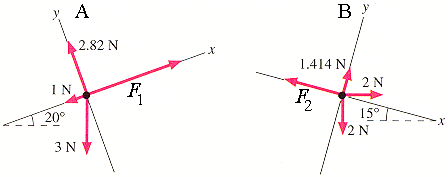# Free Body Diagrams

frownifdown
1. The question I'm struggling on has been answered on here before but with different values. Here is the link to the question https://www.physicsforums.com/showthread.php?t=344213 but on mine I have a 1.9kg object and F1=5 and F2=2.

I figured out that the Y components are 0 for both of them and I have the x component solved for F1, but I was sort of following it step by step and I'm unsure of why I did what I did. The equation used by gbednba in this example was Ax=3sin20=1.02 --> -1.02-1+5=2.97/2.6=1.14 which is the answer. I don't really understand what the 20 degree angle has to do with solving it, so if you could help me incorporate it into the problem I would appreciate that a lot. I guess I just don't really know what is going on here so if you can help me out, that would be great.

Oh and the one problem I still have to solve is for Ax of diagram B, which I don't even know what to do for.

## Answers and Replies

Homework Helper
Welcome to PF;
The values don't matter - you need to apply the method not the solution.
When you put a set of axis on your diagram, you will find that some forces are at an angle to some axis.

In the diagram on the left:the 3N force is 20deg from the -y axis - which is why the 20deg is important.
When you sum the forces in the y direction you get: ##2.82-3\cos(20^\circ)=ma_y##

See how the axis in the diagrams have been rotated to simplify the math?
In diagram A it was most convenient to rotate the axis 20deg from the usual position because that way most of the forces lined up with some axis - less trig to do.
In diagram B there was more choice - they chose a 15deg rotation to line up the force being calculated - so there are fewer steps in the calculation.

Last edited:
•1 person
frownifdown
Ah, thank you so much. So now I understand what the angle has to do with it, but I'm looking at B and am still a little stumped. When solving for the x component of the acceleration, would I take F2 (2) - 2cos(15) to get the net forces on the x axis, or do I need to figure out the x component of the 2N on the positive X axis as well and combine that (2cos15=1.93) with the F2 (-2) to get -.07 as the net forces acting on the X axis.

Sorry if my reply was confusing, it just reflects where I am right now.

Edit: Nevermind, I just figured it out! Thank you so much for all your help.

Last edited:
Homework Helper
Edit: Nevermind, I just figured it out! Thank you so much for all your help.
Well done ... just for the next person who comes along:
All the forces have to be resolved to components along the axes. It is just that the axes have been chosen so that some forces only have one non-zero component.

i.e. for B: ##\vec{F}_2 = -F_2\hat{\imath} + 0\hat{\jmath}##
Resolving to axes means that we can just use magnitudes instead of having to do a whole vector equation.
The full equation for B would go like this:

##[-F_2\hat{\imath} + 0\hat{\jmath}]+ [0\hat{\imath} + (1.414)\hat{\jmath}] + [2\cos(15^\circ)\hat{\imath} + 2\sin(15^\circ)\hat{\jmath}]+[2\sin(15^\circ)\hat{\imath} -2\cos(15^\circ) \hat{\jmath}]=m[a_x\hat{\imath} + a_y\hat{\jmath}]##

... grouping the terms in i and j gives you the familiar pair of equations.

The core trick here is to realize that the orientation of the x and y axes is totally arbitrary - y does not have to be "up" - it can point anywhere.